# Math in Focus Grade 8 Chapter 4 Lesson 4.5 Answer Key Real-World Problems: Linear Equations

Go through the Math in Focus Grade 8 Workbook Answer Key Chapter 4 Lesson 4.5 Real-World Problems: Linear Equations to finish your assignments.

## Math in Focus Grade 8 Course 3 A Chapter 4 Lesson 4.5 Answer Key Real-World Problems: Linear Equations

### Math in Focus Grade 8 Chapter 4 Lesson 4.5 Guided Practice Answer Key

Question 1.
Jeanette rents a bike while visiting a city. She pays $7 per hour to rent the bike. She also pays$8 to rent a baby seat for the bike. She pays this amount for the baby seat no matter how many hours she rents the bike. The graph shows her total cost, y dollars, after x hours.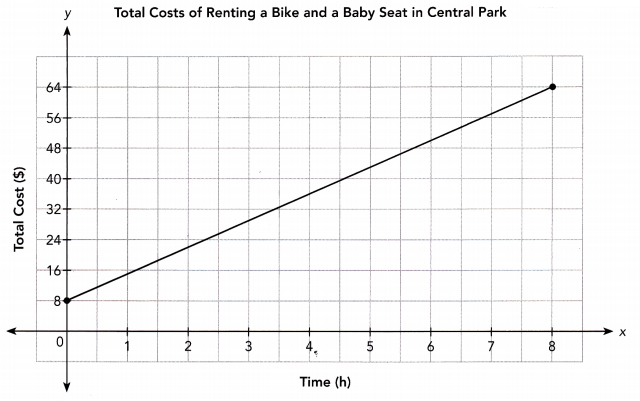a) Find the vertical intercept of the graph and explain what information it gives about the situation.
From the graph, the vertical intercept is. It represents.
From the graph, the vertical intercept is 8, It represents the initial cost of $8 to rent a baby seat for the bike. b) Find the slope of the graph and explain what information it gives about the situation. The graph passes through (,) and (,). Let (,) be (x1, y1) and (,) be (x2, y2). Slope = $$\frac{y_{2}-y_{1}}{x_{2}-x_{1}}$$ Use the slope formula. = $$\frac{?}{?}$$ Substitute values. = $$\frac{?}{?}$$ Subtract. =Simplify. The line has slope m =. It represents. Answer: From the given graph, The graph passes through the points as (0, 8) and (8, 64) Let (0, 8) be x1, y1 and (8, 64) be x2, y2. So we get the slope of the line as y2 – y1/ x2 – x1 = 64 – 8/ 8 – 0 = 56/8 = 7 Therefore, the rent charged to a bike is$7/hr.

Question 2.
Both Zack and Joy are salespeople. Each of them earns a fixed weekly salary and a percent commission based on the total sales he or she makes in a week. The graphs show the total earnings, E dollars, each person can make in one week, based on the person’s total sales, S dollars.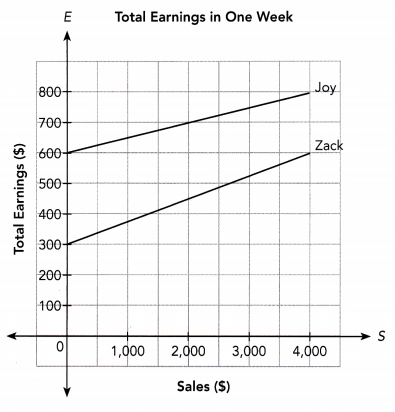a) Find the fixed weekly salary for each person.
From the graph, the vertical intercept is the fixed income of each one.
The monthly income of zack is $600 and Joy is$300.

b) Both Zack and Joy earn a percent commission. Who earns a greater percent in commission?
From the graph, Zack earns more commission
Explanation:
From the given graph,
We get the commission of Zack as,
$600 –$300 = $300 Commission of Joy as,$800 – $600 =$200
So, Zack earns more commission.

c) Find each person’s rate of commission.
From the graph,
We get each person’s commission as,
Zack’s commission as $300, Joy’s commission as$200.

Question 3.
Isaac and George are brothers who live at the same house, but go to different cities for vacation. When their vacation is over, they begin driving back home at the same time, but drive home at different speeds. Isaac’s distance D miles from their house x hours after he starts driving is given by the equation D = -50x + 150. The graph shows George’s distance D miles from their house x hours after he starts driving home.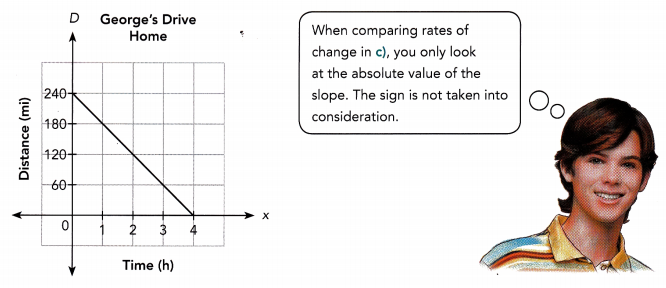a) Find the vertical intercept of George’s graph and explain what information it gives about the situation.
From the given graph, we get the vertical intercept of George’ graph as 240.
Therefore, George is 240 miles away from home.

b) Find the slope of George’s graph and explain what information it gives about the situation.
From the given graph, we get the points as (0,240) and (4,0)
We have the slope of the line as,
y2 – y1/ x2 – x1
= 0 – 240/ 4 – 0
= – 240 / 4
= -60
Here, the slope is negative which means as time increases the distance of George from his house decreases.

c) Which brother is driving faster? How do you know?
From the given graph, we get the slope as -60
The equation is given as D = -50x + 150, here the slope is -50,
So the slope for George’s graph is more, therefore he is moving faster.

### Math in Focus Course 3A Practice 4.5 Answer Key

Question 1.
Joe pays a fixed amount each month to use his cell phone. He also pays for each minute that he makes calls on the phone. The graph shows the amount, C dollars, he pays in a given month, based on the airtime, x minutes, he uses to make calls.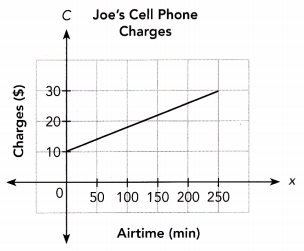a) Find the vertical intercept of the graph and explain what information it gives about the situation.
From the given graph,
We have the vertical intercept of the graph as 10,
The fixed deposit for the cell phone is $10. b) Find the slope of the graph and explain what information it gives about the situation. Answer: From the graph, we have the points as (0, 10) and (250, 30) we get the slope as, y2 – y1/ x2 – x1 = 30 – 10/ 250 – 0 = 20 / 250 = 2/25 Since slope of the curve is positive which means that when the airtime increases then the charges also increases. Question 2. Ricky and Aaron are brothers, and each of them has a coin bank. In January, the boys had different amounts of money in their coin banks. Then, for each month after that, each boy added the same amount of money to his coin bank. The graph shows the amount of savings, S dollars, in each coin bank after t months.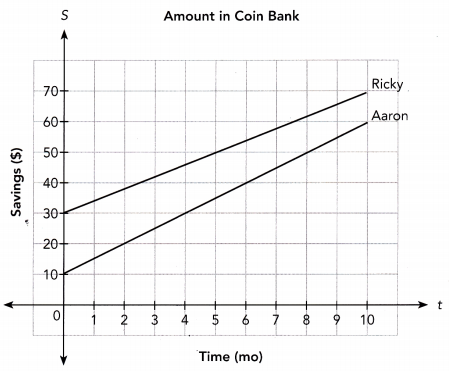a) Find the initial amount of money in each coin bank. Answer: From the graph, we get The initial amount in Ricky bank account is$30,
The initial amount in Aaron bank account is $10. b) Who added a greater amount of money each month into his coin bank? Answer: From the graph, For Aaron, the initial saving is$10 and the final saving is $60 So the money added to the bank is$50
For Ricky, the initial saving is $30 and the final saving is$70
So the money added to the bank is \$40
Therefore, Aaron added a greater amount of money each month into his coin bank.

Question 3.
Raymond and Randy drive from Town A to Town B in separate cars. The initial amount of gasoline in each car is different. The graphs show the amount of gasoline, y gallons, in each person’s car after x miles.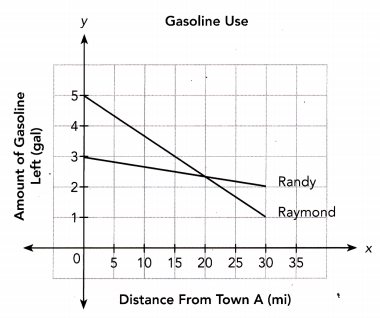a) Find the initial amount of gasoline in each car.
From the graph,
Initial amount of gasoline in Raymond is 5,
Initial amount of gasoline in Randy is 3.

b) Whose car uses more gasoline?
Given, Raymond and Randy drive from Town A and Town B in seperate cars. The graph shows the amount of gasoline, y gallons in each person’s car after x miles.
From the given graph,
Raymond’s line graph passes through the points (0, 5) and (20, 2.5)
Let (0, 5) be x1, y1 and (20, 2.5) be x2 , y2
Slope = y2 – y1 / x2 – x1
= 2.5 – 5 / 20 – 0
= – 2.5/ 20
= – 0.125
From the given graph,
Randy’s line graph passes through the points (0, 3) and (20, 2.5)
Let (0, 3) be x1, y1 and (20, 2.5) be x2, y1
Slope = y2 – y1/x2 – x1
= 2.5 – 3/ 20 – 0
= – 0.5 / 20
= – 0.025
Comparing both values, we get
Raymond’s car uses 0.125 – 0.025 = 0.1 gal of gasoline more than Randy car.

Question 4.
Pete and Winnie visit Star Cafe every day and they pay for the items using a gift card. The amount, y dollars, on Winnie’s gift card after x days is given by the equation y = 100 – 19x. The graph shows the amount on Pete’s gift card over x days.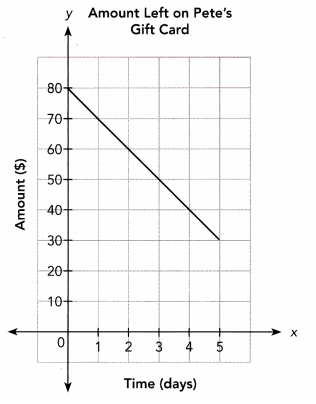a) Write an equation for the amount on Pete’s gift card.
The equation for the amount on Pete’s gift card is y = 80 – 10x
Explanation:
From the graph given,
Pete’s line graph passes through the points (0, 80) and (5, 30)
Let (0, 80) x1, y1 and (5, 30) x2, y2
Slope = y2 – y1 / x2 – x1
= 30 – 80 / 5 – 0
= – 50/ 5
= – 10
Equation of the line standard form is y – y1 = m(x – x1)
Substituting the values of slope and (x1, y1) = (0, 80) in the equation
y – 80 = – 10(x – 0)
= y – 80 = -10x + 0
= y = 80 – 10x
Hence, the equation for the amount on Pete’s gift card is y = 80 – 10x.

The amount, y dollars on Winnie’s gift card after x days is given by the equation
y = 100 – 19x — (1)
The amount, y dollars on Pete’s gift card after x days is given by the equation
y = 80 – 10x — (2)
Both the equations are in slope-intercept form
As the amount is given by vertical or y-axis, comparing the vertical interceptions of both the equations, we get
100 > 80
Therefore, Winnie’s gift card has a higher initial amount

Equation of Winnie’s gift card is given as y = 100 – 9x
We got the equation of Pete’s gift card as y = 80 – 10x
Equation of the line graphs of Winnie and Pete are given by
y = 100 – 19x — (1)
y = 80 – 10x — (2)
Can also be written as
y = -19x + 100 — (3)
y = -10x – 80 — (4)
Putting them in the slope intercept form, i.e., y = mx + b
Slope of lines Winnie’s is -19 and Pete’s is -10
Winnie spends more each day as his slope is greater.

Use graph paper. Solve.

Question 5.
A scientist attaches a spring that is 11 inches long to the ceiling and hangs weights from the spring to see how far it will stretch. The scientist records the length of the spring, y inches, for different weights x pounds.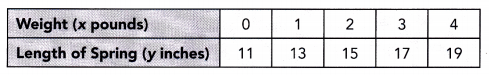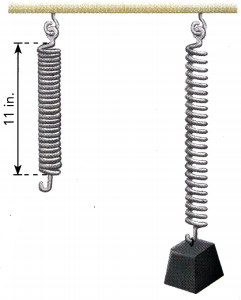a) Graph the relationship between the length of the spring for different v weights. Use 1 grid square to represent 1 unit on the horizontal axis for the x interval 0 to 4, and 1 grid square for 2 units on the vertical axis for the y interval 11 to 19.
Weight in pounds is represented by the x-axis
Length of spring in inches is represented by the y-axis
Plotting the points in the graph: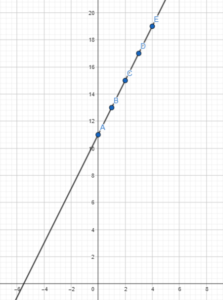b) Find the vertical intercept of the graph and explain what information it gives about the situation.
The vertical intercept of the graph is 11,
This is the amount of weight, 11 pounds.
From the graph,
The graph touches the y-axis at (0,11)c) Find the slope of the graph and explain what information it gives about the situation.
The slope of the graph is 2
It represents the rate at which the length of the spring increases for the weight in pounds,
2 inches for each pound of weight.
The graph is given byIt passes through the points (0,11) and (4,19)
Let (0,11) be x1, y1 and (4,19) be x2, y2
Slope = y2 – y1 / x2 – x1
= 19 – 11 / 4 – 0
= 8 / 4
= 2
Therefore, the slope of the graph is 2.

d) Write an equation relating the spring length and the pounds of weights hung from the spring.
The equation relating the spring stretch length and the pounds of weights hung from spring is y = 2x + 11
The graph is given byIt passes through the points (0,11) and (4,19)
Let (0,11) be x1, y1 and (4,19) be x2, y2
The slope of the graph is 2,
Substituting the values of slope and x1y1 = (0,11) in the equation, y – y1 = m(x – x1)
y – 11 = 2(x – 0)
y – 11 = 2x
y = 2x + 11
Hence, the required equation of the line is y = 2x + 11.

Brain @ Work

Question 1.
Conrad and Angeline each receive an allowance. Conrad gets the entire week’s allowance on Monday. He spends the same amount every day. Angeline gets a daily allowance starting on Monday. She saves the same amount every day. After four days, both have the same amount of money. The graph shows the amount of money, y dollars, Conrad has after x days during one week.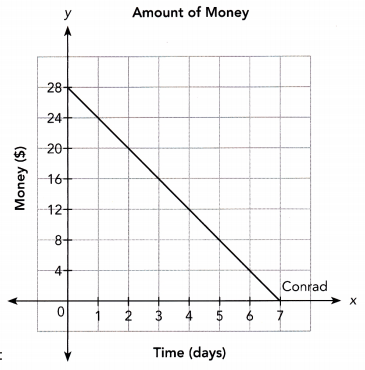a) Copy the graph. Then draw a line to represent the amount of money Angeline has after x days.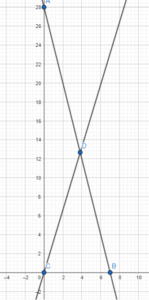The graph representing the amount of money Angeline has after d days along with that of Conrad is

b) Find the slope of Conrad’s graph and explain what information it gives about the situation.
From the above graph,
The line passes through the points (0,28) and (7,0)
Let (0,28) be x1,y1 and (7,0) be x2,y2
Slope = y2 – y1/ x2 – x1
= 0 – 28 / 7 – 0
= -28 / 7
= – 4
The slope of the graph is -4,
It represents the rate at which the amount of money of Conrad keeps on decreasing with each day. Therefore, 4 dollars for each day.

c) Write an equation to represent the amount of money each person has during that week.
The equation to represent the amount of money each person has during that week is y = 7 – x
The graph isThe slope of the line of Conrad is -4
The line passes through the points (0,28) and (7,0)
Substituting the values in equation of line,
y – y1 = m(x – x1)
=> y – 28 = -4(x – 0)
=> y = -4x + 28
=> y = -x + 28/4
=> y = -x + 7
=> y = 7 – x

Question 2.
Gordon left Townsville at 12 P.M. and started biking to Kingston 50 miles away. One and a half hours later, Jonathan left Kingston and started biking toward Townsville at a speed of 20 miles per hour. The graph shows Gordon’s distance, d miles, from Townsville after t hours.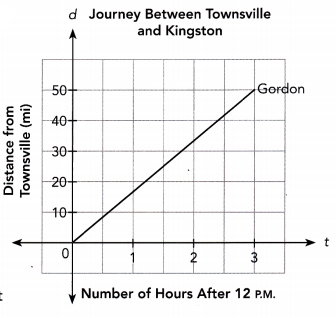a) Copy the graph. Then draw a line to represent Jonathan’s distance from Kingston after t hours.
The graph representing Jonathan’s distance from Kingston after t hours is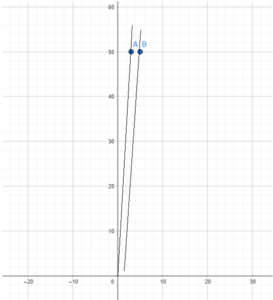From the above graph,
The line passes through the points (0,0) and (3,50)
Let (0,0) be x1,y1 and (3,50) be x2,y2
Slope = y2 – y1/ x2 – x1
= 50 – 0 / 3 – 0
= 50 / 3
= 16.67
The slope of the graph is 16.67
It represents the rate at which Gordan’s distance increases from Townsville after each hour, i.e., 16.67 miles per hour.

c) Write an equation to represent each person’s distance from Townsville after t hours.